## NCERT Exemplar Solutions for Class 10 Science Chapter 5 Periodic Classification of Elements

NCERT Exemplar Solutions for Class 10 Science Chapter 5 Multiple Choice Questions

Question 1.
Upto which element, the Law of Octaves was found to be applicable ?
(a) Oxygen
(b) Calcium
(c) Cobalt
(d) Potassium.
(b). Law was found to be applicable upto the element calcium.

More Resources

Question 2.
According to Mendeleev’s Periodic Law, the elements were arranged in the periodic table in the order of
(a) increasing atomic number
(b) decreasing atomic number
(c) increasing atomic masses
(d) decreasing atomic masses.
(c).

Question 3.
In Mendeleev’s Periodic Table, gaps were left for the elements to be discovered later. Which of the following elements found a place in the periodic table later ?
(a) Germanium
(b) Chlorine
(c) Oxygen
(d) Silicon.
(a). The element was called Eka-silicon.

Question 4.
Which of the following statement (s) about the Modern Periodic Table are incorrect ?
(i) The elements in the Modern Periodic Table are arranged on the basis of their decreasing atomic numbers
(ii) The elements in the Modern Periodic Table are arranged on the basis of their increasing atomic masses.
(iii) Isotopes are placed in adjoining group (s) in the Periodic Table
(iv) The elements in the Modern Periodic Table are arranged on the basis of their increasing atomic numbers
(a) (i) only
(b) (i), (ii) and (iii)
(c) (i), (ii) and (iv)
(d) (iv) only.
(b). Statements (i), (ii) and (iii) are all in correct.

Question 5.
Which of the following statements about the Modern Periodic Table is correct ?
(a) It has 18 horizontal rows known as Periods
(b) It has 7 vertical columns known as Periods
(c) It has 18 vertical columns known as Groups
(d) It has 7 horizontal rows known as Groups.
(c).

Question 6.
Which of the given elements A, B, C, D and E with atomic numbers 2, 3, 7, 10 and 30 respectively belong to the same period ?
(a) A, B, C
(b) B, C, D
(c) A, D, E
(d) B, D, E.
(b). The elements B (Z = 3), C (Z = 7) and D(Z = 10) belong to the same period. It is second period.

Question 7.
The elements A, B, C, D and E have atomic numbers 9, 11, 17, 12 and 13 respectively. Which pair of elements belong to the same group ?
(a) A and B
(b) B and D
(c) A and C
(d) D and E.
(c). Elements A (Z = 9) and C (Z = 17) belong to the same group. It is a halogen family (group 17).

Question 8.
Where would you locate the element with electronic configuration 2, 8 in the Modern Periodic Table ?
(a) Group 8
(b) Group 2
(c) Group 18
(d) Group 10.
(c). It is a noble gas element Neon (Ne) present in group 18.

Question 9.
An element which is an essential constituent of all organic compounds belongs to
(a) group 1
(b) group 14
(c) group 15
(d) group 16.
(b). The element is carbon. It belongs to group 14.

Question 10.
Which of the following is the outermost shell for elements of period 2 ?
(a) K shell
(b) L shell
(c) M shell
(d) N shell
(b). In second (2) period, the electrons are filled in second shell also known as L-shell.

Question 11.
Which one of the following elements exhibits maximum number of valence electrons ?
(a) Na
(b) Al
(c) Si
(d) P
(d). The element phosphorus (P) has five electrons (2, 5) in the valence shell.

Question 12.
Which of the following gives the correct increasing order of the atomic radii of O, F and N ?
(a) O, F, N
(b) N, F, O
(c) O, N, F
(d) F, O, N.
(d). It is the correct order. These elements are present in second period.

Question 13.
Which among the following elements has the largest atomic radius ?
(a) Na
(b) Mg
(c) K
(d) Ca.
(c). The element potassium (K) present in group 1 has the largest atomic radius.

Question 14.
Which of the following elements would lose an electron easily ?
(a) Mg
(b) Na
(c) K
(d) Ca.
(c). The element potassium (K) with maximum size would lose electron easily.

Question 15.
Which of the following elements does not lose an electron easily ?
(a) Na
(b) F
(c) Mg
(d) Al.
(b). The element fluorine (F) with smallest size does not lose electron easily.

Question 16.
Which of the following are the characteristics of isotopes of an element ?
(i) Isotopes of an element have same atomic mass.
(ii) Isotopes of an element have same atomic number.
(iii) Isotopes of an element show same physical properties.
(iv) Isotopes of an element show same chemical properties.
(a) (i), (iii) and (iv)
(b) (ii), (iii) and (iv)
(c) (ii) and (iii)
(d) (ii) and (iv).
(d).

Question 17.
Arrange the following elements in the order of their decreasing metallic character Na, Si, Cl, Mg, Al
(a) Cl > Si > Al > Mg > Na
(b) Na > Mg > Al > Si > Cl
(c) Na > Al > Mg > Cl > Si
(d) Al > Na > Si > Ca > Mg.
(b). The metallic character of the elements decreases along a period. These elements are present in third period.

Question 18.
Arrange the following elements in the order of their increasing non-metallic character Li, O, C, Be, F
(a) F < O < C < Be < Li
(b) Li < Be < C < O < F
(c) F < O < C < Be < Li
(d) F < O < Be < C < Li.
(b). The non-metallic character of the elements increases along a period. These elements are present in second period in the order Li, Be, C, O, F.

Question 19.
What type of oxide would Eka-aluminium form ?
(a) EO3
(b) E3O2
(c) E2O3
(d) EO.

Question 20.
Three elements B, Si and Ge are
(a) metals
(b) non-metals
(c) metalloids
(d) metal, non-metal and metalloid respectively.
(c). is the correct answer. These are also called semi-metals and possess the characteristics of both metals and non-metals.

Question 21.
Which of the following elements will form an acidic oxide ?
(a) An element with atomic number 7
(b) An element with atomic number 3
(c) An element with atomic number 12
(d) An element with atomic number 19.
(a). The element with atomic number (Z) = 7 is nitrogen. It forms acidic oxides such as N2O3, N2O5 etc.
All other elements are metals and they form basic oxides.

Question 22.
The element with atomic number 14 is hard and forms acidic oxide and a covalent halide. To which of the following categories does the element belong ?
(a) Metal
(b) Metalloid
(c) Non-metal
(d) Left-hand side element.
(b). The element with atomic number (Z) = 14 is silicon (Si). It is a metalloid.

Question 23.
Which one of the following depicts the correct representation of atomic radius (r) of an atom ?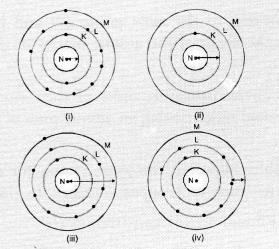(a) (i) and (ii)
(b) (ii) and (iii)
(c) (iii) and (iv)
(d) (i) and (iv).
(b). The atom (ii) has only one shell (K-shell). No electrons are present in the other shells. Therefore, the arrow represents correct atomic radius.
The arrow in atom (iii) also represents the correct atomic radius of the element.

Question 24.
Which one of the following does not increase while moving down the group of the periodic table ?
(b) Metallic character
(c) Valence
(d) Number of shells in an element.
(c). In a group, the valence or valency does not change since all the elements present have same valence shell configuration.

Question 25.
On moving from left to right in a period in the periodic table, the size of the atom
(a) increases
(b) decreases
(c) does not change appreciably
(d) first decreases and then increases
(b). Atomic size decreases along a period. However, the noble gas atom is an exception. It has very large size.

Question 26.
Which of the following set of elements is written in order of their increasing metallic character ?
(a) Be Mg Ca
(b) Na Li K
(c) Mg Al Si
(d) C O N
(a). These elements belong to group 2. These are written in increasing order of their size. Since the metallic character increases down the group, the order is the correct.

NCERT Exemplar Solutions for Class 10 Science Chapter 5 Short Answer Questions

Question 27.
The three elements A, B and C with similar properties have atomic masses X, Y and Z respectively. The mass of Y is approximately equal to the average mass of X and Z. What is such an arrangement of elements called as ? Give one example of such a set of elements.
The arrangement is known as Dobereiner’s triad. For example, Calcium (Ca), Strontium (Sr) and Barium (Ba).

Question 28.
Elements have been arranged in the following sequence on the basis of their increasing atomic masses.
F, Na, Mg, Al, Si, P, S, Cl, Ar, K
(a) Pick two sets of elements which have similar properties.
(b) The given sequence represents which law of classification of elements ?
(a) The elements that have similar properties belong to the same group. From the list of elements available, elements which belong to same group are : .
Na, K (Alkali metals) ; F, Cl (Halogens)
(b) The sequence is according to Newland’s law of octaves.

Question 29.
Can the following groups of elements be classified as Dobereiner’s triad ?
(a) Na, Si, Cl
(b) Be, Mg, Ca
Atomic mass of Be = 9; Na = 23; Mg = 24; Si = 28; Cl = 35; Ca = 40 Explain by giving reason.
(a) No, these elements cannot be classified as triads because these do not have same properties. However, the atomic mass of Si (28) is almost the mean of the atomic masses of elements Na (23) and Cl (35).
Note : The Dobereiner’s triad is meaningful only if the elements present in the triad have almost identical properties.
(b) Yes, these elements can be classified as triads. These belong to the same group (2) and have almost identical properties. The atomic mass of Mg (24) is almost the mean of the atomic masses of the elements Be (9) and Ca(40) i.e., 9 + 40 = 49/2 = 24.5.

Question 30.
In Mendeleev’s Periodic Table, the elements were arranged in the increasing order of their atomic masses. However, cobalt with atomic mass of 58.93 amu was placed before nickel having an atomic mass of 58.71 amu. Give reason for the same.
This is regarded as a defect in the Mendeleev’s periodic table. However, the main reason for making the arrangement was that the elements with similar characteristics must be grouped together. In this case,
• Cobalt (Co) should be in the company of the elements Rhodium (Rh) and Iridium (Ir).
• Nickel (Ni) should be in the company of the elements Palladium (Pd) and Platinum (Pt).

Question 31.
“Hydrogen occupies a unique position in Modern Periodic Table”. Justify the statement.
The position of the element hydrogen is still not clear even in the Modern Periodic Table.
• In electronic configuration, it resembles alkali metals of group 1. All of them have only one electron in the valence shell. Actually, hydrogen has only one shell (K-shell) which has one electron. However, it is not a metal whereas alkali-metals are metallic in nature.
• In characteristics, it resembles halogens of group 17. For example, like halogens hydrogen is a non-metal and diatomic as well.
It has been therefore, decided to assign hydrogen a unique position in the Modern or Long Form. Periodic Table. It is placed at the top in group 1 of alkali metals. However, it is not a member of that group.*

Question 32.
Write the formulae of chlorides of Eka-silicon and Eka-aluminium, the ^elements predicted by Mendeleev.
Eka-aluminium represents gallium (Ga) with valency three and Eka-silicon is for germanium (Ge) with valency four. The formulae of their respective chlorides are GaCl3 and GeCl4.

Question 33.
Three elements A, B and C have 3, 4 and 2 electrons respectively in their outermost shell. Give the group number to which they belong in the Modern Periodic Table. Also, give their valencies.
The electrons present in the outermost shell are also known as valence electrons. The desired information is given :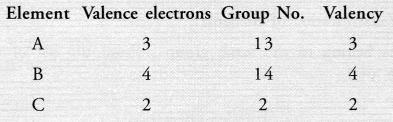Question 34.
If an element X is placed in group 14, what will be the formula and the nature of bonding of its chloride ?
The element X present in group 14 has four valence electrons in its atom. It can complete its octet by sharing four valence electrons with the electrons of other atoms. Therefore, it will form covalent bonds with the four atoms of chlorine. The formula of the chloride of the element X is.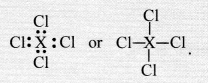Question 35.
(a) X has 12 protons and 12 electrons
(b) Y has 12 protons and 10 electrons
The available information makes it clear that :
(a) Species X with equal number of protons (12) and electrons (12) is an atom.
(b) Species Y with two electrons less (10) than the number of protons (12), is a divalent cation y2+ of species X.
Therefore, the radius of species X is more as compared to that of y2+.
Note : The radius of cation is always less than that of atom while that of anion is more.

Question 36.
Arrange the following elements in increasing order of their atomic radii.
(a) All the elements belong to the same period (second). The atomic size or radius decreases along a period. Therefore, increasing order of atomic radii is : F < N < Be < Li
(b) The listed elements are present in group 17 (halogen family). The atomic size or radius increases down
a group. Therefore, the correct increasing order of atomic radii is : Cl < Br < I < At.

Question 37.
Identify and name the metals out of the following elements whose electronic configurations are given below :
(a) 2, 8, 2
(b) 2, 8, 1
(c) 2, 8, 7
(d) 2, 1.
(a) Electronic configuration : 2, 8, 2 (Mg is a metal)
(b) Electronic configuration : 2, 8, 1 (Na is a metal)
(c) Electronic configuration : 2, 8, 7 (Cl is a non-metal)
(d) Electronic configuration : 2, 1 (Li is a metal)

Question 38.
Write the formula of the compound formed when the element A (atomic number 19) combines with the element B (atomic number 17). Draw its electronic dot structure. ^What is the nature of the bond formed ? (CBSE 2013)
Electronic configuration of element A (Z = 19) is 2, 8, 8, 1
Electronic configuration of element B(Z = 17) is 2, 8, 7.
The element A has one valence electron while the element B has seven electrons in its valence shell. One electron gets transferred from the atom of element A to the atom of element B. As a result of electron transfer, an ionic bond is formed.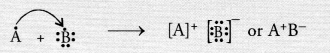Question 39.
Arrange the following elements in the increasing order of their metallic character. ,
Mg, Ca, K, Ge, Ga
In general, the metallic character increases down the group and decreases along a period. From the relative positions of the elements in the periodic table, the increasing order of metallic character is :
Ge < Ga < Mg < Ca < K.

Question 40.
Identify the elements with the following property and arrange them in increasing order of their reactivity
(a) An metal which is soft and reactive
(b) The metal which is an important constituent of limestone
(c) The metal which exists in liquid state at room temperature
(a) The metal may be either Na or K.
(b) The metal is Ca.
(c) The metal is Hg.

Question 41.
The increasing order of reactivity of the metals is :
Hg < Ca < Na < K.
Properties of the elements are given below. Where would you locate the following elements in the periodic table ?
(a) A soft metal stored under kerosene
(b) An element with variable (more than one) valency stored under water
(c) An element which is tetravalent and forms the basis of organic chemistry
(d) An element which is an inert gas with atomic number 2
(e) An element whose thin oxide layer is used to make other elements corrosion resistant by the process of “anodising.”
(a) Sodium (Group 1 and Period 3) or Potassium (Group 1 and Period 4)
(b) Phosphorus (Group 15 and Period 3). It shows variable valencies 3 and 5 and is stored under water.
(c) Carbon (Group 14 and Period 2)
(d) Helium (Group 18 and Period 1)
(e) Aluminium (Group 13 and Period 3)

NCERT Exemplar Solutions for Class 10 Science Chapter 5 Long Answer Questions

Question 42.
An element placed in 2nd group and 3rd Period of the Periodic Table, burns in the presence of oxygen to form a basic oxide.
(a) Identify the element
(b) Write the electronic configuration
(c) Write the balanced equation when it burns in the presence of air
(d) Write a balanced equation when this oxide is dissolved in water
(e) Draw the electron dot structure for the formation of this oxide
(a) The element is magnesium (Mg).
(b) The electronic configuration is 2, 8, 2.
(c) Magnesium burns in oxygen (air) to form magnesium oxide which is of basic nature.
2Mg(s) + O2(g) ————-> 2MgO(s)
(d) Magnesium hydroxide is formed.
MgO(s) + H2O(aq) ————> Mg(OH)2(s)Question 43.
An element X (atomic number 17) reacts with an element Y (atomic number 20) to form a divalent halide.
(a) Where in the Periodic Table are elements X and Y placed ?
(b) Classify X and Y as metal(s), non-metal(s) or metalloid(s).
(c) What will be the nature of oxide of element Y ? Identify the nature of bonding in the compound formed
(d) Draw the electron dot structure of the divalent halide.
Element X (Z = 17) is chlorine while element Y (Z = 20) is calcium.
(a) Chlorine (Cl) is a member of group 17 and period 3. Calcium (Ca) is present in group 2 and period 4.
(b) Chlorine is a typical non-metal while calcium is a metal.
(c) The oxide of calcium is calcium oxide (CaO). It is a basic oxide.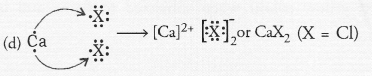Question 44.
Atomic number of a few elements are : 10, 20, 7, 14
(a) Identify the elements
(b) Identify the Group number of these elements in the Periodic Table
(c) Identify the Periods of these elements in the Periodic Table
(d) What would be the electronic configuration for each of these elements ?
(e) Determine the valency of these elements.
(a) The elements are : Neon (Z = 10), Calcium (Z = 20), Nitrogen (Z = 7) and Silicon (Z = 14)
(b) Group numbers : Neon (18), Calcium (2), Nitrogen (15), Silicon (14).
(c) Periods : Neon (2), Calcium (4), Fluorine (2), Silicon (3).
(d) Electronic Configuration : Neon (2, 8) ; Calcium (2, 8, 8, 2) Nitrogen (2, 5) ; Silicon (2, 8, 4)
(e) Valency : Neon (zero) ; Calcium (2) ; Nitrogen (3) ; Silicon (4)

Question 45.
(a) In this ladder symbols of elements are jumbled up. Rearrange these symbols of elements in the increasing order of their atomic number in the Periodic Table.
(b) Arrange them in the order of their group also.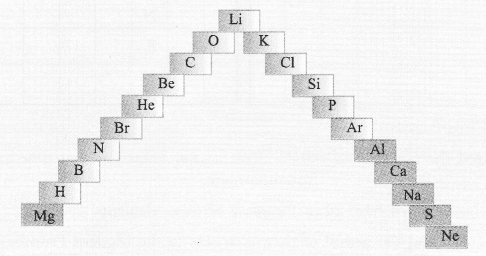(a) H, He, Li, Be, B, C, N, O, Ne, Na, Mg, Al, Si, P, S, Cl, Ar, K, Ca, Br.
(b) Group 1 — H, Li, Na, K
Group 2 — Be, Mg, Ca
Group 13 — B, A1
Group 14 — C, Si
Group 15 — N, P
Group 16 — O, S
Group 17 — Cl, Br
Group 18 — He, Ne, Ar

Question 46.
Complete the following cross word puzzle
Across :
(1) An element with atomic number 12.
(3) Metal used in making cans and member of Group 14.
(4) A lustrous non-metal which has 7 electrons in its outermost shell.
Down :
(2) Highly reactive and soft metal which imparts yellow colour when subjected to flame and is kept in kerosene.
(5) The first element of second period
(6) An element which is used in making fluorescent bulbs and is second member of group 18 in the Modern Periodic Table.
(7) A radioactive element which is the last member of the halogen family.
(8) Metal which is an important constituent of steel and forms rust when exposed to moist air.
(9) The first metalloid in Modern Periodic Table whose fibres are used in making bullet proof vests.

 1 7 2 3 8 9 5 4 6

(Cross-Puzzle)

 1M 7A G N E 2s I U M S O 3T 8I N D 9B 5L A R 4I o D I 6n E T O U R T E I N M O H O N N I N E U M

(Puzzle Solved)

Question 47.
Mendeleev predicted the existence of certain elements not known at that time and named two of them as Eka-silicon and Eka-aluminium.
(a) Name the elements which have taken the place of these elements.
(b) Mention the group and the period of these elements in the Modern Periodic Table.
(c) Classify these elements as metals, non-metals or metalloids.
(d) How many valence electrons are present in each one of them ?
(a) Germanium (Ge) for Eka-silicon and gallium (Ga) for Eka-aluminium
(b) Germanium (Group 14 and Period 4)
Gallium (Group 13 and Period 4)
(c) Germanium (Metalloid) ; Gallium (Metal)
(d) Germanium (Z = 32), Four valence electrons (2, 8, 18, 4)
Gallium (Z = 31) ; Three valence electrons (2, 8, 18, 3).

Question 48.
(a) Electropositive nature of the element(s) increases down the group and decreases across the period
(b) Electronegativity of the element decreases down the group and increases across the period
(c) Atomic size increases down the group and decreases across a period (left to right)
(d) Metallic character increases down the group and decreases across a period.
On the basis of the above trends of the Periodic Table, answer the following about the elements with atomic numbers 3 to 9.
(a) Name the most electropositive element among them.
(b) Name the most electronegative element.
(c) Name the element with smallest atomic size.
(d) Name the element which is a metalloid.
(e) Name the element which shows maximum valency.
(a) Lithium (Z = 3) is the most electropositive element,
(b) Fluorine (Z = 9) is the most electronegative element.
(c) Fluorine (Z = 9) has the smallest atomic size.
(d) Boron (Z = 5) is a metalloid.
(e) Carbon (Z = 6) shows the maximum valency (4). However, the element nitrogen (Z = 7) can show valency 5 in some compounds (e.g., N2O5).

Question 49.
An element X which is a yellow solid at room temperature shows catenation and allotropy. X forms two oxides which are also formed during the thermal decomposition of ferrous sulphate crystals and are the major air pollutants.
(a) Identify the element X.
(b) Write the electronic configuration of X.
(c) Write the balanced chemical equation for the thermal decomposition of ferrous sulphate crystals.
(d) What would be the nature (acidic/basic) of oxides formed ?
(e) Locate the position of the element in the Modern Periodic Table.
(a) The available information suggests that the element X is sulphur.
(b) Electronic configuration of S(Z = 16) 2, 8, 6(d) Fe2O3 (basic oxide), SO2(acidic oxide), SO3(acidic oxide)
(e) Sulphur is a member of group 16 and period 3.

Question 50.
An element X of group 15 exists as diatomic molecule and combines with hydrogen at 773 K in presence of the catalyst to form a compound, ammonia which has a characteristic pungent smell.
(a) Identify the element X. How many valence electrons does it have ?
(b) Draw the electron dot structure of the diatomic molecule of X. What type of bond is formed in it ?
(c) Draw the electron dot structure for ammonia. What type of bonds is formed in it ?
(a) The available information suggests that the element X is nitrogen (N) and exists in diatomic form as N,
Electronic configuration of N(Z = 7) ; 2, 5. It has five valence electrons.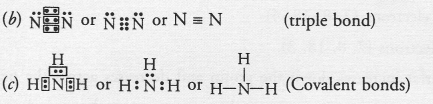Question 51.
Which group of elements could be placed in Mendeleev’s Periodic Table without disturbing the original order ? Give reason.
Group of noble gases (called zero group) could be placed in the Mendeleev’s Periodic Table with out disturbing the original order.
Reasons : Elements present in a group have same valency in the Mendeleev’s Periodic Table. Based on their electronic configuration, the members of noble gas family have zero valency. That is why they are called inert gases. They could be easily placed in the Mendeleev’s Periodic Table as a separate group without disturbing the arrangement of other elements.

Question 52.
Give an account of the process adopted by Mendeleev for the classification of elements. How did he arrive at “Periodic Law” ?